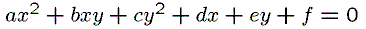Java Applet

## Quadratic curve is determined by 5 pointsrepresents ellipse,hyperbola,parabola.
(exceptionally,2 lines,1 line,1 point etc)

If the curve doesn't pass origine,then we can suppose f=1.

Next if we specify 5 points to pass,then values of a,b,c,d,e determine. So we obtain one quadratic curve.

In this applet you can move 5 points and draw corresponding quadratic curve.

[Home] [Geometric toy] [Polyhedral toy] [Java Applet] [Links] [What's New]